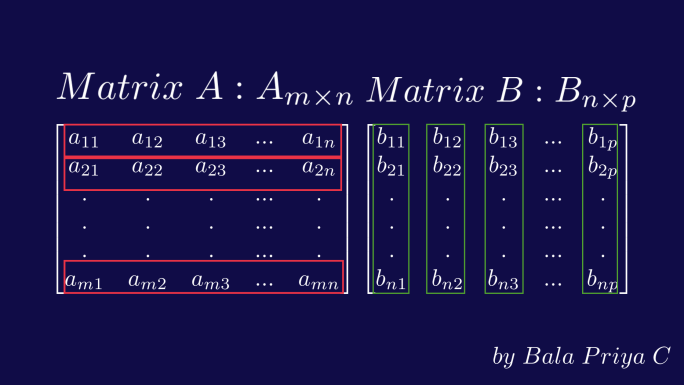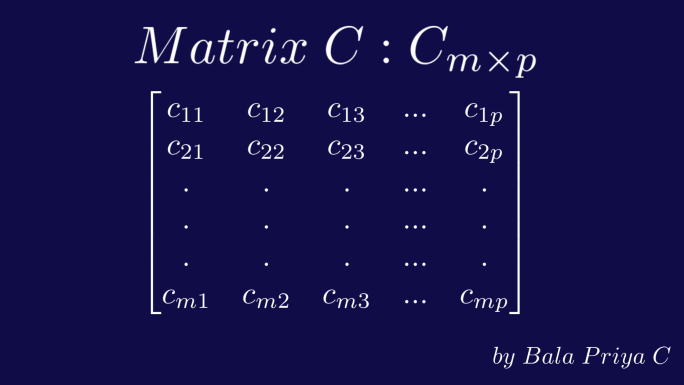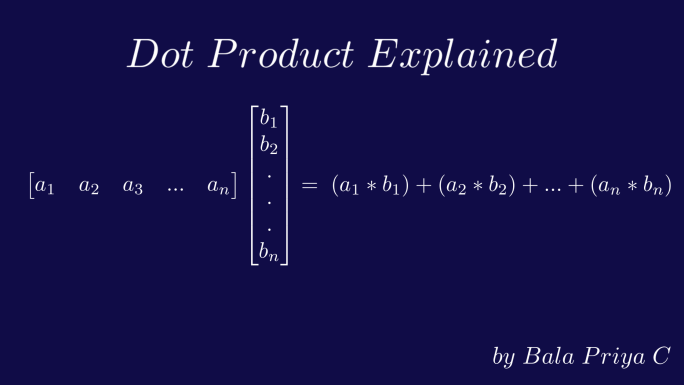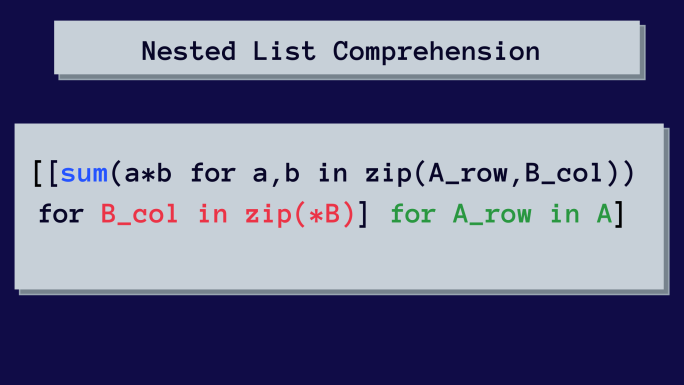Sunday, December 3, 2023
HomeTech3 Ways to Multiply Matrices in Python

# 3 Ways to Multiply Matrices in Python

This article will teach you how to multiply two matrices using Python. You will then develop a custom Python function to multiply matrices. Using nested list comprehensions, we will now demonstrate how to achieve the same outcome. You will then utilize NumPy and its built-in functions to execute matrix multiplication more efficiently.

## How to Verify the Validity of Matrix Multiplication

Before building Python code for matrix multiplication, let’s review matrix multiplication’s fundamentals. Matrix Multiplication between two matrices A and B is only valid if the number of columns in A equals the number of rows in B.

You have probably seen this condition for matrix multiplication previously. Have you ever questioned why this is the case? This is because of how matrix multiplication operates. Examine the image displayed below. In our example, matrix A consists of m rows and n columns. In addition, matrix B contains n rows and p columns.

##What is the Product Matrix’s Shape?

The element located at index I j) in the resulting matrix C is the dot product of row I of matrix A and column j of matrix B. To obtain an element at a specific index in the resultant matrix C, you must compute the dot product of the respective row and column in matrices A and B. By repeating the above steps, the product matrix C will have m rows and p columns, as shown below.The following equation describes the dot product or the inner product between two vectors a and b.Now let’s summarize:

• Clearly, the dot product is only defined between vectors of identical length.
• When multiplying two matrices, for the dot product between a row and a column to be valid, both matrices must have the same amount of elements.
• In the preceding example, each row of matrix A has n elements. And each column in matrix B also has n elements.

Upon closer inspection, n represents the number of columns in matrix A and the number of rows in matrix B. And this is precisely why the number of columns in matrix A must correspond to the number of rows in matrix B. I trust that you comprehend the criterion for valid matrix multiplication and how to acquire each element of the product matrix. Let’s proceed to multiply two matrices with Python code.

## 1. Create a Python Function to Perform Matrix Multiplication

Let’s begin by creating a custom function to multiply matrices. This function should perform the tasks below:

• Accept as inputs the matrices A and B.
• Verify that the multiplication of matrices A and B is correct.
• Multiply the two matrices A and B, if valid, then return the product matrix C.
• Return an error message if the A and B matrices cannot be multiplied.

Step 1: Generate two integer matrices using the random.randint() method in NumPy. You can also declare matrices as Python lists with nesting.

``````import numpy as np
np.random.seed(27)
A = np.random.randint(1,10,size = (3,3))
B = np.random.randint(1,10,size = (3,2))
print(f"Matrix A:\n {A}\n")
print(f"Matrix B:\n {B}\n")

# Output
Matrix A:
[[4 9 9]
[9 1 6]
[9 2 3]]

Matrix B:
[[2 2]
[5 7]
[4 4]]``````

Step 2: Proceed to define the multiply matrix function (A,B). If matrix multiplication is legitimate, this function accepts two matrices A and B as inputs and returns the product matrix C.

``````def multiply_matrix(A,B):
global C
if  A.shape == B.shape:
C = np.zeros((A.shape,B.shape),dtype = int)
for row in range(rows):
for col in range(cols):
for elt in range(len(B)):
C[row, col] += A[row, elt] * B[elt, col]
return C
else:
return "Sorry, cannot multiply A and B."``````

### Parsing Function Definition

Let’s parse the function definition now.

Declare C a global variable: And they are inaccessible outside the function. To make the product matrix C outside available, we must define it as a global variable. Simply prepend the global qualifier to the variable’s name.

Check the validity of matrix multiplication: Use the shape attribute to determine whether or not A and B can be multiplied. arr.shape and arr.shape provide the number of rows and columns, respectively, for any array arr. Therefore, if A.shape == B.shape verifies the validity of matrix multiplication. Only if this condition is satisfied can the product matrix be calculated. The function returns an error message otherwise.

Utilize nested loops for value computation: The outer for loop traverses the rows of matrix A to calculate the elements of the resultant matrix. The inner for loop allows us to traverse each column of the matrix B. And the innermost for loop facilitates access to each column element.

Now that we understand how the Python code to multiply matrices operates, let’s call the function with the previously constructed matrices A and B.

``````multiply_matrix(A,B)

# Output
array([[ 89, 107],
[ 47,  49],
[ 40,  44]])``````

As multiplication of matrices A and B is valid, the multiply matrix() function returns the resultant matrix C.

## 2. Apply Python Nested List Comprehension to Matrix Multiplication

In the preceding part, you created a Python function for matrix multiplication. Now you will see how to perform the same thing with nested list comprehensions. Here is the layered list comprehension for matrix multiplication.This may initially appear complex. However, we will step by step parse the hierarchical list comprehension. Let’s identify the purpose of one list comprehension at a time. The following will serve as a general pattern for list comprehension:

``````[<do-this> for <item> in <iterable>]

where,
<do-this>: what you'd like to do—expression or operation
<item>: each item you'd like to perform the operation on
<iterable>: the iterable (list, tuple, etc.) that you're looping through``````

Refer to our List Comprehension in Python – with Examples guide for a comprehensive understanding. Before proceeding, please note that the resultant matrix C will be constructed one row at a time.

### List Comprehension Explanation

Step 1: compute a single value in matrix C. Given the row I of matrix A and column j of matrix B, the expression below returns the entry at the index I j) of matrix C.

``````sum(a*b for a,b in zip(A_row, B_col)

# zip(A_row, B_col) returns an iterator of tuples
# If A_row = [a1, a2, a3] & B_col = [b1, b2, b3]
# zip(A_row, B_col) returns (a1, b1), (a2, b2), and so on``````

If I = j = 1, the expression will return the matrix C item c 11. Thus, you can obtain a single element in a single row.

Step 2: Construct one row in matrix C

Our next objective is to construct a full row.

For row 1 in matrix A, it is necessary to iterate through each column in matrix B to obtain a complete row in matrix C.

• Replace do-this> with the expression from step 1, as this is the desired result.
• Replace item> with B col, which represents each column in matrix B.
• Replace iterable> with zip(*B) — the list of all columns in matrix B.

The first list comprehension follows.

``````[sum(a*b for a,b in zip(A_row, B_col)) for B_col in zip(*B)]

# zip(*B): * is the unzipping operator
# zip(*B) returns a list of columns in matrix B``````

Step 3: Construct each row and produce matrix C

Next, you must complete the product matrix C by calculating the remaining rows.

Consequently, you must iterate across each row in matrix A.

Return to the list comprehension yet again and complete the steps below.

• Replace do-this’ with the list comprehension established in step 2. Recall that one complete row was computed in the previous phase.
• Replace item> with A row, which represents each row in matrix A.
• And your iterable is the matrix A itself, since you are iterating over its rows.

This concludes our discussion of layered list comprehension.

``````[[sum(a*b for a,b in zip(A_row, B_col)) for B_col in zip(*B)]
for A_row in A]``````

Time to confirm the result!

``````# cast into <a href="https://geekflare.com/numpy-reshape-arrays-in-python/">NumPy array</a> using np.array()
C = np.array([[sum(a*b for a,b in zip(A_row, B_col)) for B_col in zip(*B)]
for A_row in A])

# Output:
[[ 89 107]
[ 47  49]
[ 40  44]]``````

Upon closer inspection, this is comparable to the nested for loops we had previously, albeit shorter. This can also be accomplished more effectively by utilizing several in-built capabilities. Learn about them in the following section.

## 3. Matrix Multiplication with NumPy matmul() in Python

The np.matmul() function accepts two matrices as input and returns the product if matrix multiplication is valid.

``````C = np.matmul(A,B)
print(C)

# Output:
[[ 89 107]
[ 47  49]
[ 40  44]]``````

Observe how this method is simpler than the two previously taught methods. In reality, you may replace np.matmul() with an equivalent @ operator, as we’ll see immediately.
How to Use the Python @ Operator to Multiply Matrices

### How to Use @ Operator in Python to Multiply Matrices

It acts on two matrices and, in general, NumPy arrays with N dimensions, and returns the product matrix.

Note: Python 3.5 or later is required to use the @ operator.

Here’s how to utilize it.

``````C = A@B
print(C)

# Output
array([[ 89, 107],
[ 47,  49],
[ 40,  44]])``````

Observe that the product matrix C is identical to the one we received previously.

## Can np.dot() be Used to Multiply Matrices?

If you’ve ever encountered code that multiplies two matrices using np.dot(), this is how it works.

``````C = np.dot(A,B)
print(C)

# Output:
[[ 89 107]
[ 47  49]
[ 40  44]]``````

You will notice that np.dot(A, B) produces the anticipated product matrix as well.

Nevertheless, according to the NumPy docs, np.dot() should only be used to compute the dot product of two one-dimensional vectors and not for matrix multiplication.

Remember that the element at index I j) of the product matrix C is the dot product of the row I of matrix A and the column j of matrix B.

As a result of NumPy’s implicit broadcasting of the dot product operation to all rows and columns, you obtain the product matrix. Instead, use np.matmul() or the @ operator to make your code more understandable and avoid ambiguity.

## Conclusion

This tutorial has taught you the following. For matrix multiplication to be legitimate, the number of columns in matrix A must equal the number of rows in matrix B.
How to create a Python function that verifies the validity of matrix multiplication and returns the product matrix. The function’s body contains nested for loops.
Next, you learnt how to multiply matrices using layered list comprehensions. They are more concise than for loops but can have readability problems.
Finally, you learnt how to multiple matrices using the NumPy built-in function np.matmul() and how this is the fastest method.
You also learnt how to multiply two matrices in Python using the @ operator.

Our discussion on matrix multiplication in Python concludes here. Learn how to determine whether an integer is prime in Python as the following stage. Or, solve intriguing problems involving Python strings.